# Community

Blog Events Webinars Tutorials Forum
×
Community Blog Train and Deploy an Image Classification Model Using MacOS

# Train and Deploy an Image Classification Model Using MacOS

This article explains how to train and deploy an image classification model using MacOS.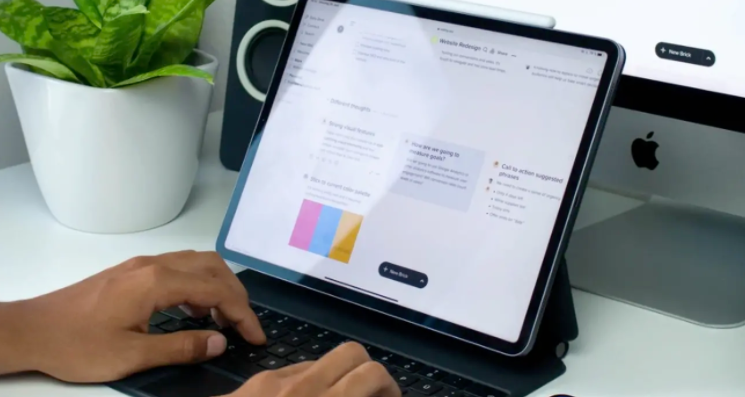By Suchuan from F(x) Team

Some of you may be confused and do not know how to get started when studying machine learning. You have read various algorithms introduced in classic books, but you still do not know how to use them to solve problems. After learning the algorithms, you need to prepare environments and train and deploy machines. It is very troublesome.

Today, I would like to introduce an easy-to-use method and provide ready-made samples and codes. Following the steps in this article, you can experience the whole process of using machine learning with MacOS.

In the following demo, the final effect is that the classification of a given image can be predicted. For example, the samples we use to train the model are cats and dogs, meaning the model can learn about cats and dogs. If the training samples are buttons and search boxes, the model can learn those buttons and search boxes.

If you want to know how to use machine learning to solve practical problems, you can read an article entitled, How to Use Deep Learning to Recognize UI Elements? It describes problem definition, algorithm selection, sample preparation, model training, model evaluation, model service deployment, and model application.

## Environment Preparation

### Installing Anaconda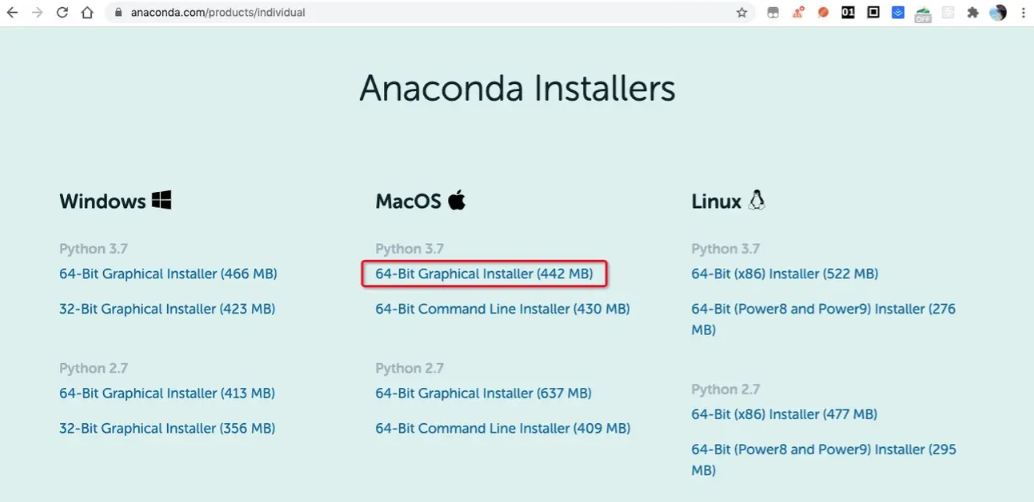After the installation, you can run the following command on the terminal command line to bring environment variables into effect immediately:

``\$ source ~/.bashrc``

You can run the following command to view environment variables:

``\$ cat ~/.bashrc``

You can see that environment variables of Anaconda have been automatically added to the .bashrc file.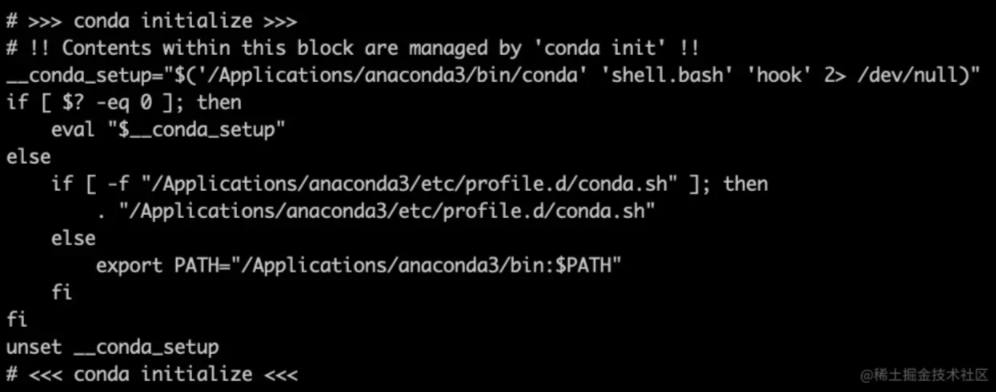Run the following command:

``\$ conda list``

You can see that many packages have been installed in Anaconda. If you use these packages, you do not need to install them again. A Python environment is also installed.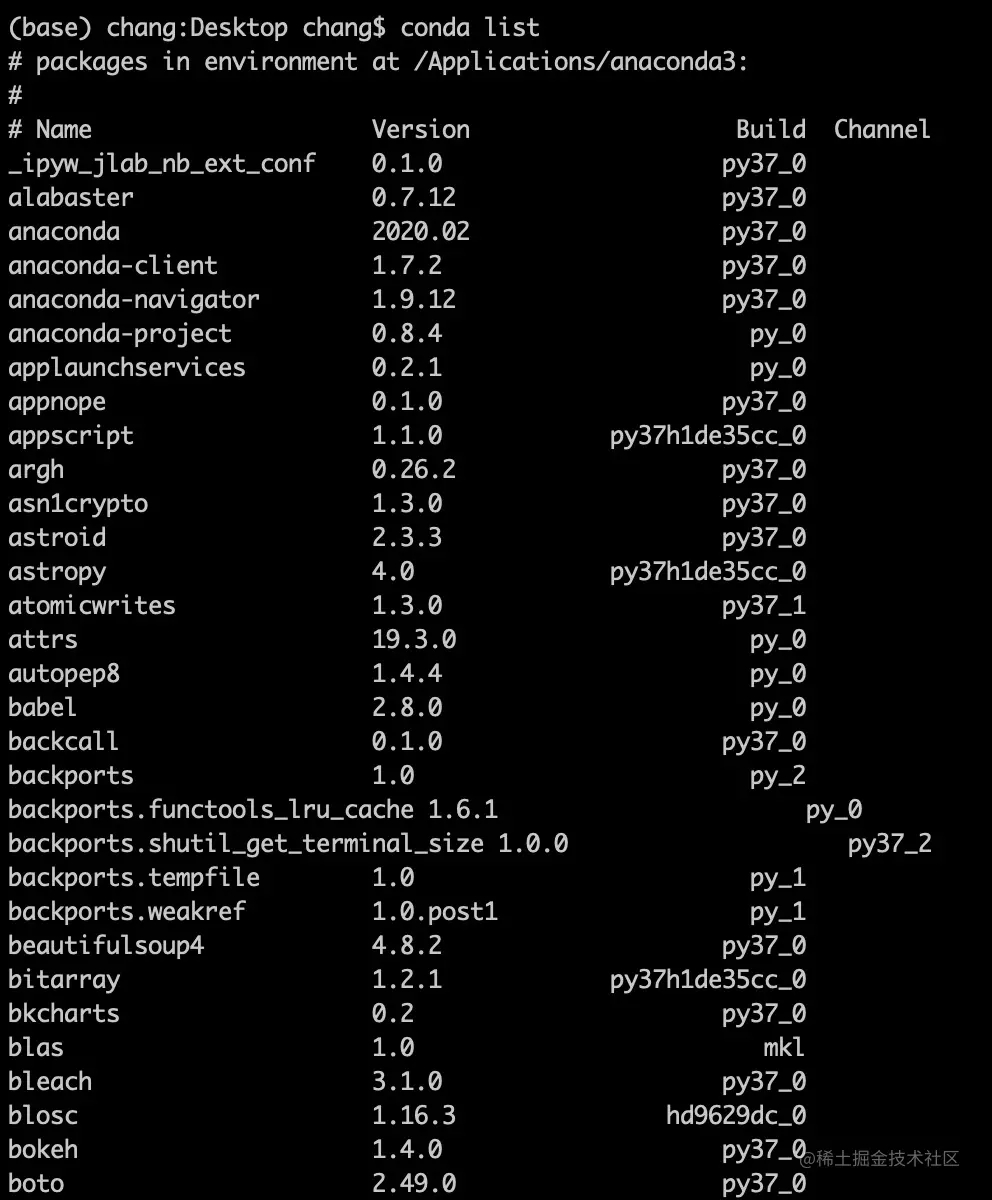Note: If the installation fails, reinstall it. When indicated where to install, click Change Installation Location. It will not select the installation location by default. You can choose where to install it and put it under Application.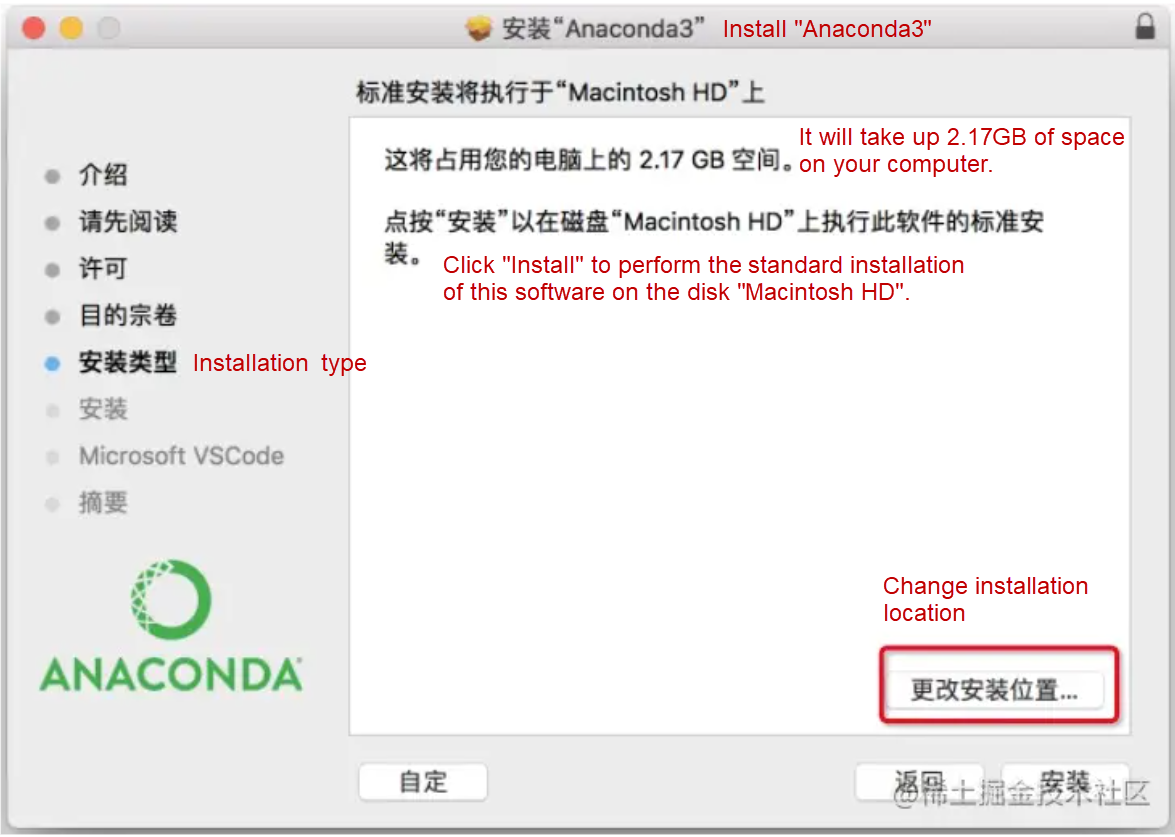### Installing Related Dependencies

Anaconda does not contain Keras, TensorFlow, or OpenCV-Python. You need to install them separately.

``````\$ pip install keras
\$ pip install tensorflow
\$ pip install opencv-python``````

## Sample Preparation

Only four classifications are prepared here: Button, Keyboard, Searchbar, and Switch. Each one contains about 200 samples.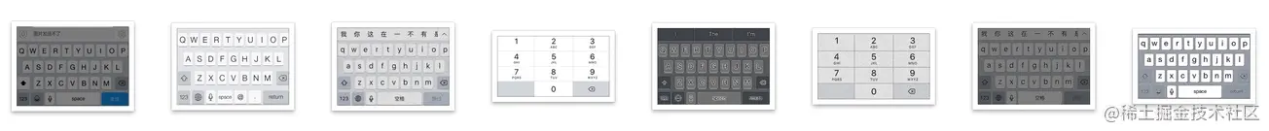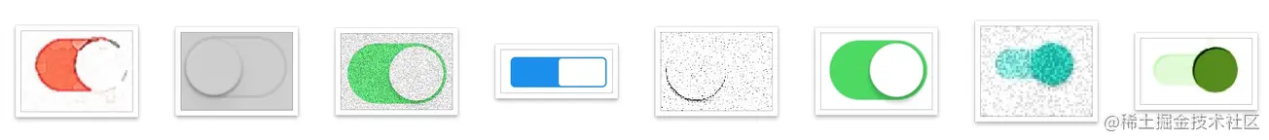## Model Training

### Developing and Training Logic

Create a project named train-project. The file structure is listed below:

``````.
├── CNN_net.py
├── dataset
├── nn_train.py
└── utils_paths.py``````

The entry file code is listed below. The logic is to enter prepared samples into the image classification algorithm, SimpleVGGNet, and set some training parameters, such as learning rate, Epoch, and Batch Size. After executing this training logic, you will finally get a model file.

``````# nn_train.py
from CNN_net import SimpleVGGNet
from sklearn.preprocessing import LabelBinarizer
from sklearn.model_selection import train_test_split
from sklearn.metrics import classification_report

from keras.optimizers import SGD
from keras.preprocessing.image import ImageDataGenerator
import utils_paths
import matplotlib.pyplot as plt
from cv2 import cv2
import numpy as np
import argparse
import random
import pickle

import os

data = []
labels = []

# Obtain the image data path for subsequent reading
imagePaths = sorted(list(utils_paths.list_images('./dataset')))
random.seed(42)
random.shuffle(imagePaths)

image_size = 256
for imagePath in imagePaths:
image = cv2.resize(image, (image_size, image_size))
data.append(image)
label = imagePath.split(os.path.sep)[-2]
labels.append(label)

data = np.array(data, dtype="float") / 255.0
labels = np.array(labels)

# Split data set
(trainX, testX, trainY, testY) = train_test_split(data,labels, test_size=0.25, random_state=42)

# Convert tags to the one-hot encoding format
lb = LabelBinarizer()
trainY = lb.fit_transform(trainY)
testY = lb.transform(testY)

# Data enhancement processing
aug = ImageDataGenerator(
rotation_range=30,
width_shift_range=0.1,
height_shift_range=0.1,
shear_range=0.2,
zoom_range=0.2,
horizontal_flip=True,
fill_mode="nearest")

# Establish a convolutional neural network
model = SimpleVGGNet.build(width=256, height=256, depth=3,classes=len(lb.classes_))

# Set initialization hyper-parameters

# Learning rate
INIT_LR = 0.01
# Epoch
# Setting at 5 here is to finish training as soon as possible. You can set it higher, such as 30
EPOCHS = 5
# Batch Size
BS = 32

# Use the loss function, and compile the model
print("------Start training network------")
opt = SGD(lr=INIT_LR, decay=INIT_LR / EPOCHS)
model.compile(loss="categorical_crossentropy", optimizer=opt,metrics=["accuracy"])

# Train network model
H = model.fit_generator(
aug.flow(trainX, trainY, batch_size=BS),
validation_data=(testX, testY),
steps_per_epoch=len(trainX) // BS,
epochs=EPOCHS
)

# Test
print("------Test network------")
predictions = model.predict(testX, batch_size=32)
print(classification_report(testY.argmax(axis=1), predictions.argmax(axis=1), target_names=lb.classes_))

# Draw the result curve
N = np.arange(0, EPOCHS)
plt.style.use("ggplot")
plt.figure()
plt.plot(N, H.history["loss"], label="train_loss")
plt.plot(N, H.history["val_loss"], label="val_loss")
plt.plot(N, H.history["accuracy"], label="train_acc")
plt.plot(N, H.history["val_accuracy"], label="val_acc")
plt.title("Training Loss and Accuracy")
plt.xlabel("Epoch #")
plt.ylabel("Loss/Accuracy")
plt.legend()
plt.savefig('./output/cnn_plot.png')

# Save the model
print("------Save the model------")
model.save('./cnn.model.h5')
f = open('./cnn_lb.pickle', "wb")
f.write(pickle.dumps(lb))
f.close()``````

If the data set is large for practical application scenarios, Epoch will also be set to be large, and the data set will be trained on a high-performance machine. If you want to complete training tasks on MacOS, we only provide a few samples to train the model. Epoch is also very small (Epoch=5). The recognition accuracy of the model will be very poor, but this article explains how to complete a machine learning task on MacOS.

### Starting Training

Run the following command to start training:

``\$ python nn_train.py``

The training process logs are shown below: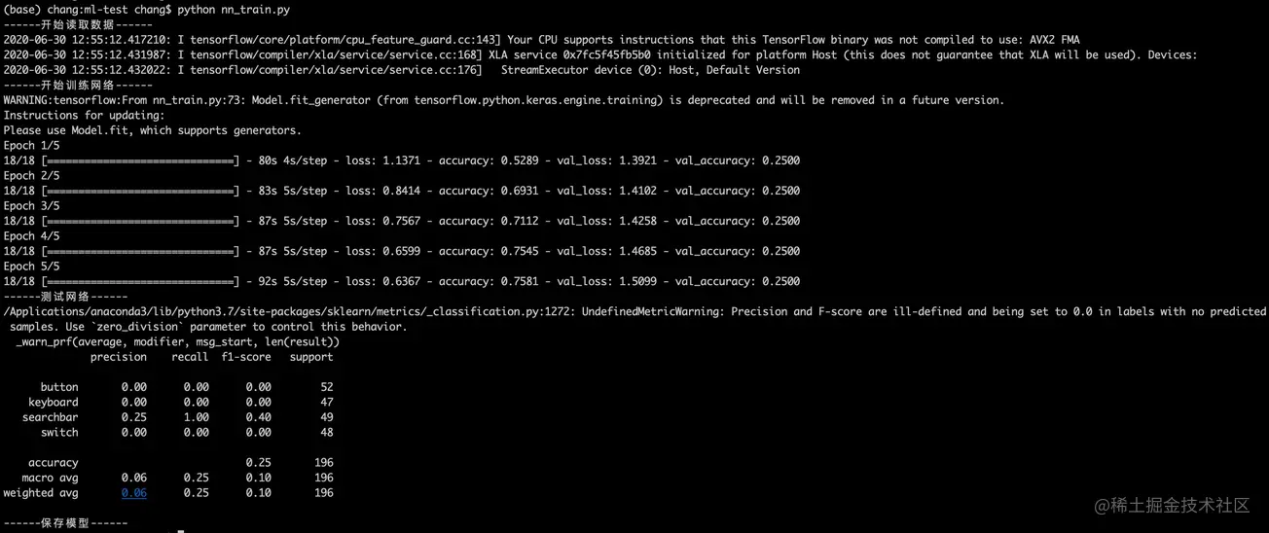After training, two files are generated in the current directory: model file, cnn.model.h5, and the curve of loss function, output/cnn_plot.png: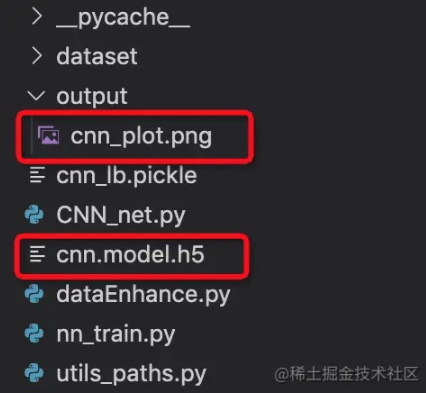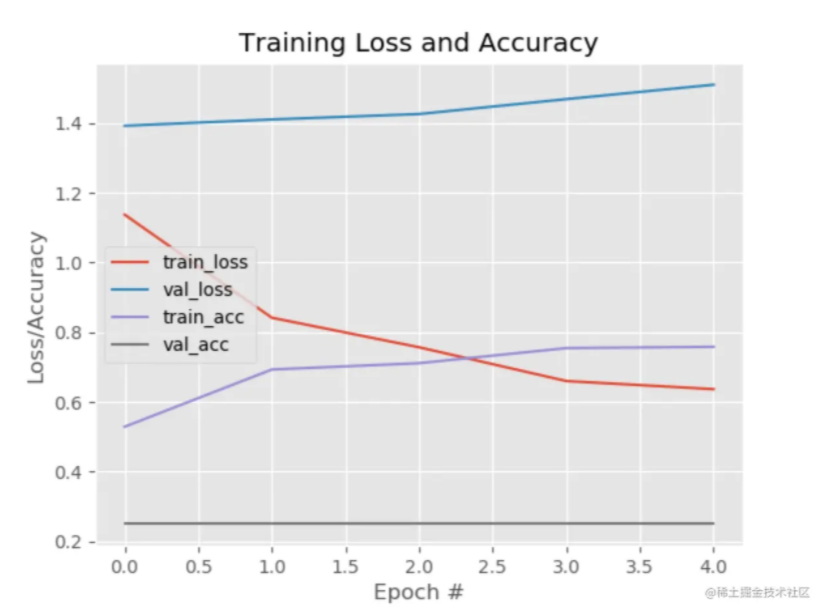## Model Evaluation

Since we have the model file named cnn.model.h5, we can write a prediction script and execute the script locally to predict the classification of images:

``\$ python predict.py``
``````# predict.py
import allspark
import io
import numpy as np
import json
from PIL import Image
import requests
import cv2
import os
import tensorflow as tf
import time

# The input of pred should be an array of images, and the images have been converted into the format of NumPy arrays.
# pred = model.predict(['./validation/button/button-demoplus-20200216-16615.png'])

# This order must be as same as the order of label.json. The model output is an array, and the maximum value index is set as the predicted value.
Label = [
"button",
"keyboard",
"searchbar",
"switch"
]
testPath = "./test/button.png"

images = []
image = cv2.cvtColor(image, cv2.COLOR_BGR2RGB)

image = cv2.resize(image,(256,256))
images.append(image)
images = np.asarray(images)

pred = model.predict(images)

print(pred)

max_ = np.argmax(pred)
print('Prediction result:',Label[max_])``````

If you want to know the accuracy of this model, you can also enter a batch of data with known classifications into the model. After the model prediction, you can compare the classification predicted by the model with the actual classification to calculate the accuracy rate and recall rate.

## Deployment of Model Service

### Develop Model Service

However, we predict the classification of an image in practical application by requesting an API to get the return result through a given image. We need to write a model service and deploy it to the remote end to get a deployed model service API.

Now, we can write a model service and deploy it locally.

``````# Model service app.py
import allspark
import io
import numpy as np
import json
from PIL import Image
import requests
import cv2
import tensorflow as tf

with open('label.json') as f:
labels = {value:key for key,value in mp.items()}

def create_opencv_image_from_stringio(img_stream, cv2_img_flag=-1):
img_stream.seek(0)
image_temp = cv2.imdecode(img_array, cv2_img_flag)
if image_temp.shape == 4:
image_channel3 = cv2.cvtColor(image_temp, cv2.COLOR_BGRA2BGR)
return image_channel3
else:
return image_temp

def get_string_io(origin_path):
r = requests.get(origin_path, timeout=2)
stringIo_content = io.BytesIO(r.content)
return stringIo_content

def handleReturn(pred, percent, msg_length):
result = {
"content":[]
}
argm = np.argsort(-pred, axis = 1)
for i in range(msg_length):
label = labels[argm[i, 0]]
index = argm[i, 0]
if(pred[i, index] > percent):
confident = True
else:
confident = False
result['content'].append({'isConfident': confident, 'label': label})
return result

def process(msg, model):
percent = msg_dict['threshold']
msg_dict = msg_dict['images']
msg_length = len(msg_dict)
desire_size = 256

images = []
for i in range(msg_length):
image_temp = create_opencv_image_from_stringio(get_string_io(msg_dict[i]))
image_temp = cv2.cvtColor(image_temp, cv2.COLOR_BGR2RGB)
image = cv2.resize(image_temp, (256, 256))
images.append(image)
images = np.asarray(images)
pred = model.predict(images)
return bytes(json.dumps(handleReturn(pred, percent, msg_length)) ,'utf-8')

while True:
try:
rsp = process(msg, model)
srv.write(rsp)
except Exception as e:
srv.error(500,bytes('invalid data format', 'utf-8'))

if __name__ == '__main__':
desire_size = 256

context = allspark.Context(4)
queued = context.queued_service()

workers = []
for i in range(10):
t.setDaemon(True)
t.start()
workers.append(t)
for t in workers:
t.join()``````

### Deploy Model Service

After the model service is written, it requires an installation environment to be deployed locally. First, create a model service project, deploy-project, copy the cnn.model.h5 to this project, and install the environment in this project.

``````.
├── app.py
├── cnn.model.h5
└── label.json``````

### Install Docker

Mac Docker Installation Document

``````# Installing Docker with Homebrew requires to install Homebrew first: https://brew.sh

After the installation, the Docker icon will appear on the desktop.

### Create Virtual Environment for Anaconda

``````# Use conda to create a python environment. The directory needs to be named ENV
\$ conda create -p ENV python=3.7

# Install the EAS python sdk
\$ ENV/bin/pip install http://eas-data.oss-cn-shanghai.aliyuncs.com/sdk/allspark-0.9-py2.py3-none-any.whl

# Install other dependency packages
\$ ENV/bin/pip install tensorflow keras opencv-python

# Activate the virtual environment
\$ conda activate ./ENV

# Exit the virtual environment (when it is out of use)
\$ conda deactivate``````

### Run Docker Environment

/Users/chang/Desktop/ml-test/deploy-project

Replace it with your own project path:

``````sudo docker run -ti -v  /Users/chang/Desktop/ml-test/deploy-project:/home -p 8080:8080
registry.cn-shanghai.aliyuncs.com/eas/eas-python-base-image:py3.6-allspark-0.8``````

### Local Deployment

Now, you can deploy locally after running the following command:

``````cd /home
./ENV/bin/python app.py``````

The following log shows the deployment is successful.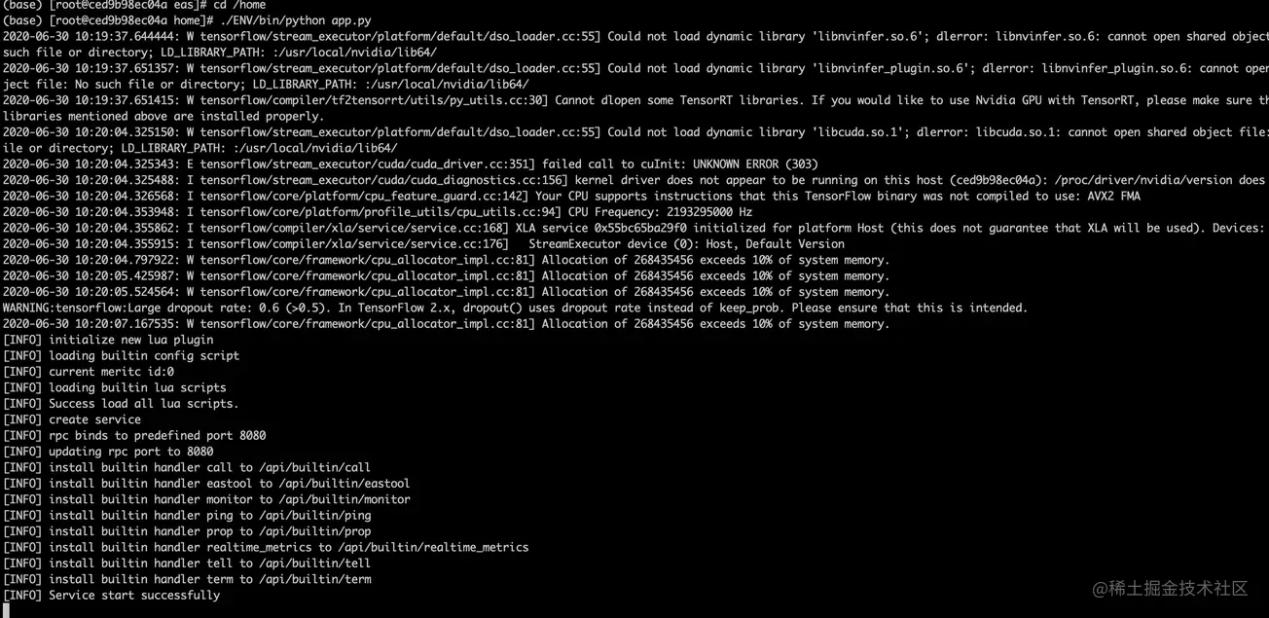After deployment, you can access the model service by localhost:8080/predict.

You can use the curl command to send a post request to predict the classification of an image:

``````curl -X POST 'localhost:8080/predict' \
-H 'Content-Type: application/json' \
-d '{
"images": ["https://img.alicdn.com/tfs/TB1W8K2MeH2gK0jSZJnXXaT1FXa-638-430.png"],
"threshold": 0.5
}'``````

Obtain the prediction result:

``{"content": [{"isConfident": true, "label": "keyboard"}]}``

## Complete Code

You can clone code the repository via this link.

After installing environments, you can run the following command:

``````# 1、Train the model
\$ cd train-project
\$ python nn_train.py

# Generate a model file: cnn.model.h5

# 2、Copy the model file to the deploy-project and deploy the model service
# Install the runtime environment for model service first
\$ conda activate ./ENV
\$ sudo docker run -ti -v  /Users/chang/Desktop/ml-test/deploy-project:/home -p 8080:8080  registry.cn-shanghai.aliyuncs.com/eas/eas-python-base-image:py3.6-allspark-0.8
\$ cd /home
\$ ./ENV/bin/python app.py

# Obtain the model service API: localhost:8080/predict

# 3、Access the model service
curl -X POST 'localhost:8080/predict' \
-H 'Content-Type: application/json' \
-d '{
"images": ["https://img.alicdn.com/tfs/TB1W8K2MeH2gK0jSZJnXXaT1FXa-638-430.png"],
"threshold": 0.5
}'``````

## Summary

Let's summarize the process of using deep learning. We choose SimpleVGGNet as the image classification algorithm (which is equivalent to a function), transmit the prepared data to this function, and run this function (to learn the characteristics and labels of the dataset) to get an output result, which is the model file ` model.h5`.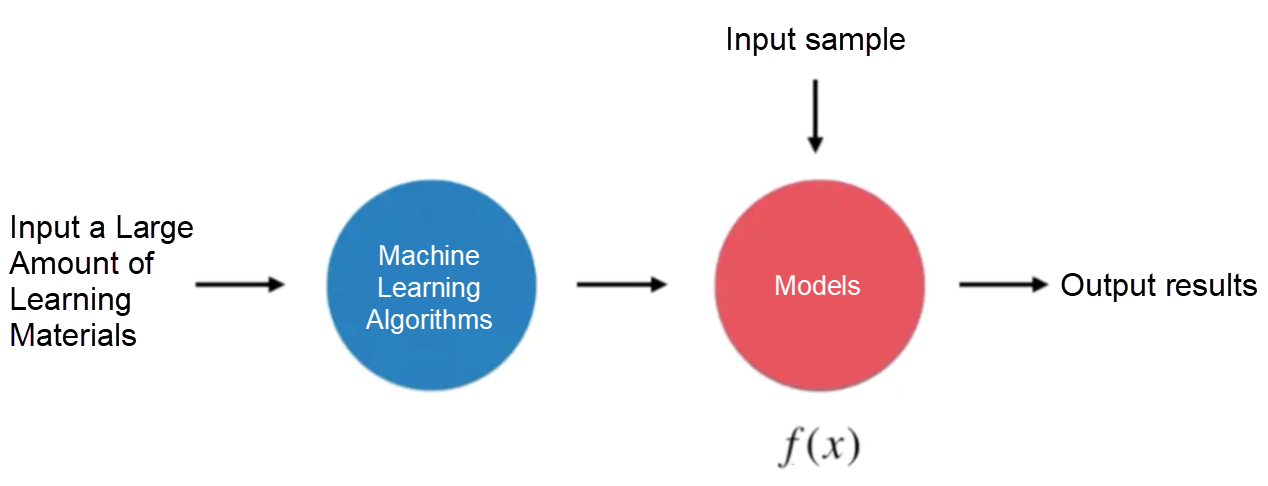This model file can receive an image as input, predict what the image is, and output the prediction result. However, if you want the model to run online, you need to write a model service (API) and deploy it online to get an HTTP API, which we can call directly in the production environment.

0 0 0
Share on

# Related Products

• ##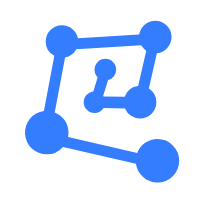Machine Learning Platform For AI

An end-to-end platform that provides various machine learning algorithms to meet your data mining and analysis requirements.

• ##Epidemic Prediction Solution

This technology can be used to predict the spread of COVID-19 and help decision makers evaluate the impact of various prevention and control measures on the development of the epidemic.

• ##Online Education Solution

This solution enables you to rapidly build cost-effective platforms to bring the best education to the world anytime and anywhere.

• ##Accelerated Global Networking Solution for Distance Learning

Alibaba Cloud offers an accelerated global networking solution that makes distance learning just the same as in-class teaching.